# Lines Parallel to the Same Line

Lines Parallel to the Same Line

Consider a line l, and suppose that we draw two more lines m and n such that l $$\parallel$$ m and l $$\parallel$$ n.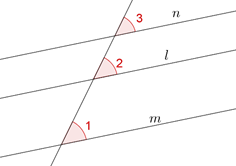Can we say that m $$\parallel$$ n? Draw any transversal across the three lines, as we have done above, and note that

$$\angle 1$$ = $$\angle 2$$ (corresponding angles)

$$\angle 2$$ = $$\angle 3$$ (corresponding angles)

Thus,

$$\angle 1$$ = $$\angle 3$$

This proves that m must be parallel to n. We formalize this result in the form of a theorem.

Theorem: Two or more lines which are parallel to the same line will be parallel to each other.

Consider a line l, and consider two more lines m and n such that l $$\parallel$$ m and l $$\parallel$$ n, as shown below: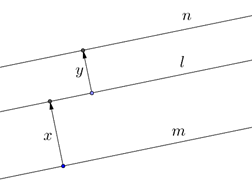The distance between l and m is x, and the distance between l and n is y. What is the distance between m and n? The theorem above tells us that m and n will also be parallel, and therefore there will be a fixed (constant) distance between them. Clearly, that distance will be x + y.

Example 1: Consider the following figure, in which L1 and L2 are parallel lines: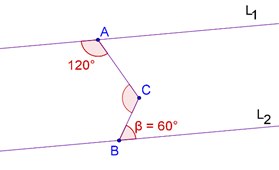What is the value of $$\angle C$$?

Solution: Through C, draw a line parallel to L1 and L2, as shown below: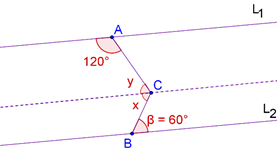We have:

$$\angle x$$ = $$\angle \beta$$ = 600 (alternate interior angles)

$$\angle y$$ = 1800 – 1200 (co-interior angles)

è $$\angle y$$ = 600

Thus,

$$\angle C$$ = $$\angle x$$ + $$\angle y$$ = 1200

Example 2: Consider the following figure, in which L1, L2 and L3 are parallel lines. The line AC is perpendicular to L3, as shown: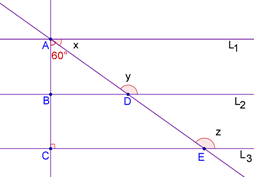What are the values of $$\angle x$$, $$\angle y$$ and $$\angle z$$?

Solution: Since AC ⊥ L3, it will be perpendicular to each of the other two parallels as well. This means that:

$$\angle x$$ = 900 – 600 = 300

Now,

$$\angle y$$ = 1800 – $$\angle x$$ (co-interior angles)

è $$\angle y$$ = 1500

è $$\angle z$$ = $$\angle y$$ = 1500 (corresponding angles)

Example 3: Consider the following figure, in which L1 $$\parallel$$ L2: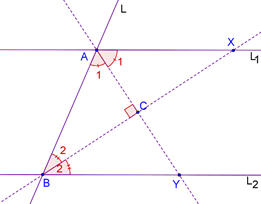The angle bisectors of $$\angle BAX$$ and $$\angle ABY$$ intersect at C, as shown. Prove that $$\angle ACB$$ = 900, as indicated in the figure.

Solution: We note that $$\angle BAX$$ and $$\angle ABY$$ are co-interior angles, which means that their sum is 1800:

$$\angle BAX$$ + $$\angle ABY$$ = 1800

è 2($$\angle 1$$ + $$\angle 2$$) = 1800

è $$\angle 1$$ + $$\angle 2$$ = 900

Now, in ΔACB, we apply the angle sum property:

è $$\angle 1$$ + $$\angle 2$$ + $$\angle ACB$$ = 1800

è 900 + $$\angle ACB$$ = 1800

è $$\angle ACB$$ = 900

This completes our proof.

Example 4: Consider the following figure, in which AX $$\parallel$$ CY. What is the value of $$\angle B$$?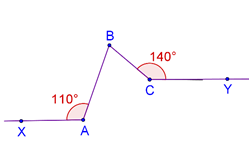Solution: Through A and C, drawn two lines perpendicular to AX and CY. Through B, draw a line parallel to these perpendiculars, as shown (the three dotted lines are parallel):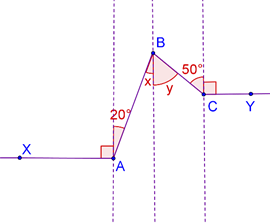Note how we have marked the various angles. Clearly,

$$\angle x$$ = 200 (alternate interior angles)

$$\angle y$$ = 500 (alternate interior angles)

Thus,

$$\angle B$$ or $$\angle ABC$$ = $$\angle x$$ + $$\angle y$$ = 700.

Example 5: Consider the following figure, in which AX $$\parallel$$ DY: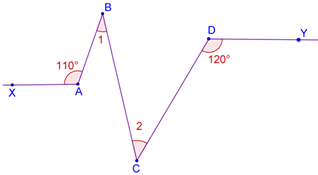What is the difference between $$\angle 1$$ and $$\angle 2$$?

Solution: Through A and D, draw perpendiculars to AX and DY. Through B and C, draw parallels to these perpendiculars, as shown below (note that the four dotted lines are parallel to each other):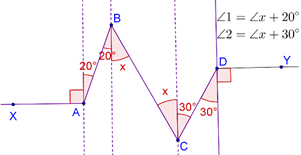Carefully note how we have marked the various angles, using the fact that alternate interior angles for parallel lines are equal. Now, we have:

$$\angle 1$$ = $$\angle x$$ + 200

$$\angle 2$$ = $$\angle x$$ + 300

Thus,

$$\angle 2$$ – $$\angle 1$$ = ($$\angle x$$ + 300) – ($$\angle x$$ + 200) = 100

Note that this difference is the same as the difference of $$\angle CDY$$ and $$\angle BAX$$. That is, the actual values of $$\angle B$$ and $$\angle D$$ have no effect on their difference.

Example 6: Three parallel lines are intersected by a transversal, as shown below: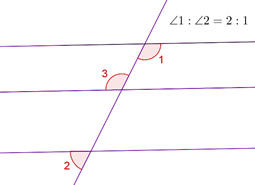If $$\angle 1$$:$$\angle 2$$ = 2:1, what is the value of $$\angle 3$$?

Solution: Consider the following figure: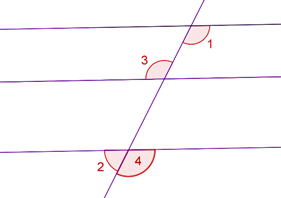Clearly, $$\angle 1$$ = $$\angle4$$, because these are corresponding angles. Now,

$$\angle 1$$:$$\angle 2$$ = 2:1 è $$\angle4$$:$$\angle 2$$ = 2:1

Also, $$\angle4$$ + $$\angle 2$$ = 1800, because they are a linear pair. Using these two facts, it is easy to conclude that:

$$\angle4$$ = 1200, $$\angle 1$$ = 600

Thus, $$\angle 1$$ = $$\angle4$$ = 1200, and hence,

$$\angle 3$$ = $$\angle 1$$ = 1200 (alternate interior angles)

Geometry
grade 9 | Questions Set 1
Geometry
Geometry
grade 9 | Questions Set 2
Geometry
More Important Topics
Numbers
Algebra
Geometry
Measurement
Money
Data
Trigonometry
Calculus
More Important Topics
Numbers
Algebra
Geometry
Measurement
Money
Data
Trigonometry
Calculus